# Venn diagram

(diff) ← Older revision | Latest revision (diff) | Newer revision → (diff)

A graphic representation of formulas of mathematical logic, mainly formulas of the propositional calculus. A Venn diagram ofvariablesof classical propositional logic is a selection of closed contours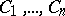(with homeomorphic circumferences) which subdivides the plane intodomains, some of which (e.g.,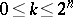) are marked. Each marked domain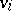,, is put into correspondence with the formula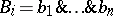where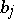,, is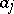iflies within the contour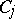andisotherwise. The formula corresponding to the diagram as a whole is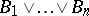. Thus, the Venn diagram in the figure corresponds to the formulaIf there are no marked domains (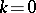), the diagram corresponds to an identically-false formula, e.g.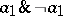. In propositional logic, Venn diagrams are used to solve decision problems, the problem of deducing all possible pairwise non-equivalent logical consequences from given premises, etc. Propositional logic may be constructed as operations over Venn diagrams brought into correspondence with logical operations.Figure: v096550a

The apparatus of diagrams was proposed by J. Venn  to solve problems in the logic of classes. The method was then extended to the classical many-place predicate calculus. Venn diagrams are used in applications of mathematical logic and theory of automata, in particular in solving the problems of neural nets.

How to Cite This Entry:
Venn diagram. Encyclopedia of Mathematics. URL: http://encyclopediaofmath.org/index.php?title=Venn_diagram&oldid=12932
This article was adapted from an original article by A.S. Kuzichev (originator), which appeared in Encyclopedia of Mathematics - ISBN 1402006098. See original article